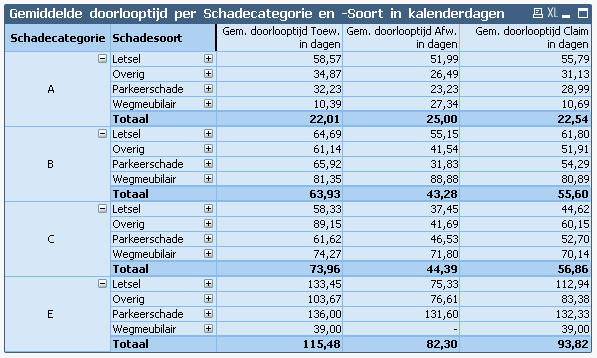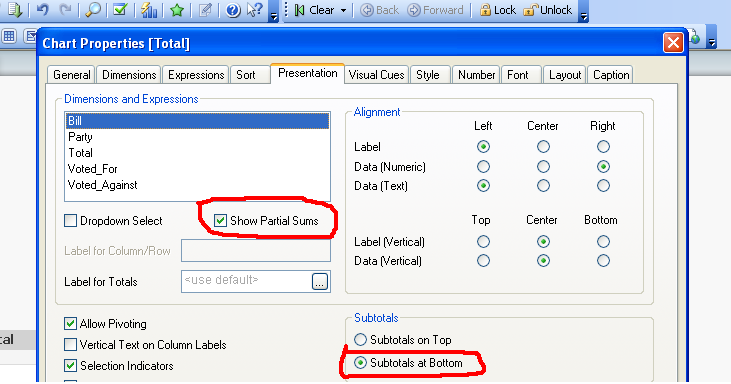New to QlikView

Discussion board where members can get started with QlikView.

Announcements
Highlighted
Contributor III

How to add general totals on average expressions in a pivot table?

I have this pivot table, which shows average values. I derived these values from a database table, by substracting creation date from decision date (in a damage claim), which renders throughput time in a damage claim (time from creation to decision).

In my database table, I created three extra extra values: Throughput time for negative and positive decisions and one for 'total' throughput time (which of course equals one of the former two, per record in my database).

In my pivot table, I want to show AVERAGE throughput time per claim category and type. I used avg() functions as three expressions.

Somehow, I can't totalise throughput time for ALL categories and types....

I am sure the solution should be simple, but ticking the boxes for subtotals for my dimensions did NOT do it...

Here's the table:Tags (2)
1 Solution

Accepted Solutions
Contributor III

Re: How to add general totals on average expressions in a pivot table?

I guess I have finally found the answer....

When using an 'If' clause in an expression, QV won't totalize that expression in a (pivot) table. Once I got rid of that clause, it summed my table flawlessly...

8 RepliesMVP & Luminary

Re: How to add general totals on average expressions in a pivot table?

I hope this would be helpful for you: Average – Which average?

- Marcus

Contributor III

Re: How to add general totals on average expressions in a pivot table?

Thanks, Marcus! But.. it's not about calculating average throughput times, although the suggestions on that page sure are helpful, but about getting those averages summed up and displayed as a general total (weighed) average..MVP & Luminary

Re: How to add general totals on average expressions in a pivot table?

I'm not quite sure what you want to be calculated and how the result should looks like but mosten often you need to calculate rather sum(y) / count(y) instead of avg(y) and/or you need an aggr-functions which wrapped one of those calculations like: avg(aggr(sum(y) / count(y), Dimensions)) or sum(aggr(avg(y), Dimensions)) and you could also use different calculations for the different chart-levels if you queried them per if(dimensionality() < 1, exp1, exp2).

- MarcusNot applicable

Re: How to add general totals on average expressions in a pivot table?

Hi,

Go For Presentation tab,as do as shown int the below image,for which dimension you want the total select that and click on show partial sum as shown int the below figure.Contributor III

Re: How to add general totals on average expressions in a pivot table?

Hi Harshitha,

I wished it was that simple... Those boxes are already ticked....Contributor III

Re: How to add general totals on average expressions in a pivot table?

I would like - below the box 'E' to be a box named 'Total', which shows average totals per row for the damage types and a grand total.MVP & Luminary

Re: How to add general totals on average expressions in a pivot table?

Like above mentioned you could enable the partial sums for Schadecategorie and use a aggr() or an dimensionality-condition to calculate your wanted values. If they are very special it could be that you need to create within the script an additionally dummy-value Totaal for Schadecategorie and then react with an if-loop in your calculation if it's a real value for the expression or your dummy whereby the calculation then might be extend with some set analysis statement and/or total qualifier.

- Marcus

Contributor III

Re: How to add general totals on average expressions in a pivot table?

I guess I have finally found the answer....

When using an 'If' clause in an expression, QV won't totalize that expression in a (pivot) table. Once I got rid of that clause, it summed my table flawlessly...